# bteve documentation¶

bteve is a Python driver for BridgeTek’s EVE series GPUs. In particular it supports the Gameduino 3X series of display adapters.

It supports:

• Python running on Windows/MacOS/Linux, connected via a SPIDriver to the Gameduino or BT81x

• CircuitPython on an embedded board, including

• Adafruit M4 Metro and Feather

• Teensy 4.x

• Raspberry Pi Pico

import sys
import bteve as eve

if sys.implementation.name == "circuitpython":
gd = eve.Gameduino()
else:
from spidriver import SPIDriver
gd = eve.Gameduino(SPIDriver(sys.argv))
gd.init()

gd.ClearColorRGB(0x20, 0x40, 0x20)
gd.Clear()
gd.cmd_text(gd.w // 2, gd.h // 2, 31, eve.OPT_CENTER, "Hello world")
gd.swap()import sys
import random
import bteve as eve

rr = random.randrange

if sys.implementation.name == "circuitpython":
gd = eve.Gameduino()
else:
from spidriver import SPIDriver
gd = eve.Gameduino(SPIDriver(sys.argv))
gd.init()

while True:
gd.Clear()
gd.Begin(eve.POINTS)
for i in range(100):
gd.ColorRGB(rr(256), rr(256), rr(256))
gd.PointSize(rr(100))
gd.Vertex2f(rr(gd.w), rr(gd.h))
gd.swap()# Module classes¶

## Gameduino¶

The Gameduino class is a specialization of the base class EVE.

class Gameduino([d])
Parameters

d (spidriver) – when running on a PC, a SPIDriver object for communicating with the EVE hardware

init()

Initialize the EVE hardware. Confirm that the BT81x is running, configure it for the attached screen, and render a blank frame.

On CircuitPython this method uses cpy:sdcardio to attach to the GD3X microSD card as "/sd/", so any files on the card can be accessed with the prefix "/sd/".

w

Width of the display, in pixels. Available after calling init().

h

Height of the display, in pixels. Available after calling init().

rd(a, n)

Parameters
• a (int) – address in EVE memory

• n (int) – number of bytes to read

Return bytes

memory contents

wr(a, bb)

Write directly to EVE memory

Parameters
• a (int) – address in EVE memory

• bb (bytes) – bytes to write

rd32(a)

Read a 32-bit value from EVE memory :param int a: address in EVE memory :returns int: memory contents

wr32(a, v)

Write a 32-bit value to EVE memory

Parameters
• a (int) – address in EVE memory

• v (int) – value to write

is_finished()

Returns True if the EVE command FIFO is empty

Returns bool

True if the EVE command FIFO is empty

This method is the non-blocking equivalent of EVE.finish().

result(n=1)
Returns int

result field

Return the result field of the most recent command, if any.

## EVE¶

This class provides all graphics drawing operations, graphics state operations, and graphics commands.

Methods for simple drawing and drawing state:

Methods for clearing the screen:

Methods to set the 2D scissor clipping rectangle:

Methods to set the tag state, so that touch events can be attached to screen objects:

Methods to preserve and restore the graphics state:

Methods to control rendering and display:

Methods to set the alpha blend state, allowing more advanced transparency and compositing operations:

Methods to set the stencil state, allowing conditional drawing and other logial operations:

Low-level methods to set the bitmap format (See cmd_setbitmap() for a higher-level alternative.):

Low-level methods set the bitmap transform matrix (See cmd_scale(), cmd_translate(), cmd_setmatrix() etc. for a higher-level alternative.):

Methods to set the precision and offset used by Vertex2f():

• VertexTranslateX()

• VertexTranslateY()

• VertexFormat()

class EVE
AlphaFunc(func, ref)

Set the alpha test function

Parameters

These values are part of the graphics context and are saved and restored by SaveContext() and RestoreContext().

Begin(prim)

Begin drawing a graphics primitive

Parameters

prim (int) – graphics primitive.

Examples

def zigzag(title, x):
for i in range(3):
gd.Vertex2f(x - 14,   25 + i * 90)
gd.Vertex2f(x + 14,   25 + 45 + i * 90)
gd.cmd_text(x, 0, 27, eve.OPT_CENTERX, title)

gd.Begin(eve.BITMAPS)
zigzag("BITMAPS", 48)

gd.Begin(eve.POINTS)
zigzag("POINTS",  48 + 1 * 96)

gd.Begin(eve.LINES)
zigzag("LINES",  48 + 2 * 96)

gd.Begin(eve.LINE_STRIP)
zigzag("LINE_STRIP",  48 + 3 * 96)

gd.Begin(eve.RECTS)
zigzag("RECTS",  48 + 4 * 96)BitmapExtFormat(format)

Set the bitmap format

Parameters

format (int) – bitmap pixel format.

BitmapHandle(handle)

Set the bitmap handle

Parameters

handle (int) – bitmap handle. Range 0-31. The initial value is 0

This value is part of the graphics context and is saved and restored by SaveContext() and RestoreContext().

BitmapLayout(format, linestride, height)

Set the source bitmap memory format and layout for the current handle

Parameters
• format (int) – bitmap pixel format, or GLFORMAT to use BITMAP_EXT_FORMAT instead. Range 0-31

• linestride (int) – bitmap line stride, in bytes. Range 0-1023

• height (int) – bitmap height, in lines. Range 0-511

BitmapLayoutH(linestride, height)

Set the source bitmap memory format and layout for the current handle. high bits for large bitmaps

Parameters
• linestride (int) – high part of bitmap line stride, in bytes. Range 0-7

• height (int) – high part of bitmap height, in lines. Range 0-3

BitmapSize(filter, wrapx, wrapy, width, height)

Set the screen drawing of bitmaps for the current handle

Parameters
BitmapSizeH(width, height)

Set the screen drawing of bitmaps for the current handle. high bits for large bitmaps

Parameters
• width (int) – high part of drawn bitmap width, in pixels. Range 0-3

• height (int) – high part of drawn bitmap height, in pixels. Range 0-3

Set the source address for bitmap graphics

Parameters

addr (int) – Bitmap start address, pixel-aligned. May be in SRAM or flash. Range 0-16777215

BitmapSwizzle(r, g, b, a)

Set the source for the r,g,b and a channels of a bitmap

Parameters
• r (int) – red component source

• g (int) – green component source

• b (int) – blue component source

• a (int) – alpha component source

The source parameter may be one of:

BitmapTransformA(p, v)

Set thecomponent of the bitmap transform matrix

Parameters
• p (int) – precision control: 0 is 8.8, 1 is 1.15. Range 0-1. The initial value is 0

• v (int) – Thecomponent of the bitmap transform matrix, in signed 8.8 or 1.15 bit fixed-point form. Range 0-131071. The initial value is 256

The initial value is p = 0, v = 256. This represents the value 1.0.

These values are part of the graphics context and are saved and restored by SaveContext() and RestoreContext().

BitmapTransformB(p, v)

Set the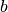component of the bitmap transform matrix

Parameters
• p (int) – precision control: 0 is 8.8, 1 is 1.15. Range 0-1. The initial value is 0

• v (int) – Thecomponent of the bitmap transform matrix, in signed 8.8 or 1.15 bit fixed-point form. Range 0-131071. The initial value is 0

The initial value is p = 0, v = 0. This represents the value 0.0.

These values are part of the graphics context and are saved and restored by SaveContext() and RestoreContext().

BitmapTransformC(v)

Set the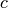component of the bitmap transform matrix

Parameters

v (int) – Thecomponent of the bitmap transform matrix, in signed 15.8 bit fixed-point form. Range 0-16777215. The initial value is 0

This value is part of the graphics context and is saved and restored by SaveContext() and RestoreContext().

BitmapTransformD(p, v)

Set thecomponent of the bitmap transform matrix

Parameters
• p (int) – precision control: 0 is 8.8, 1 is 1.15. Range 0-1. The initial value is 0

• v (int) – Thecomponent of the bitmap transform matrix, in signed 8.8 or 1.15 bit fixed-point form. Range 0-131071. The initial value is 0

The initial value is p = 0, v = 0. This represents the value 0.0.

These values are part of the graphics context and are saved and restored by SaveContext() and RestoreContext().

BitmapTransformE(p, v)

Set the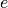component of the bitmap transform matrix

Parameters
• p (int) – precision control: 0 is 8.8, 1 is 1.15. Range 0-1. The initial value is 0

• v (int) – Thecomponent of the bitmap transform matrix, in signed 8.8 or 1.15 bit fixed-point form. Range 0-131071. The initial value is 256

The initial value is p = 0, v = 256. This represents the value 1.0.

These values are part of the graphics context and are saved and restored by SaveContext() and RestoreContext().

BitmapTransformF(v)

Set the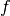component of the bitmap transform matrix

Parameters

v (int) – Thecomponent of the bitmap transform matrix, in signed 15.8 bit fixed-point form. Range 0-16777215. The initial value is 0

This value is part of the graphics context and is saved and restored by SaveContext() and RestoreContext().

BlendFunc(src, dst)

Set pixel arithmetic

Parameters

These values are part of the graphics context and are saved and restored by SaveContext() and RestoreContext().

Examples

gd.Begin(eve.POINTS)
gd.ColorRGB(0xf8, 0x80, 0x17)
gd.PointSize(160)
gd.BlendFunc(eve.SRC_ALPHA, eve.ONE_MINUS_SRC_ALPHA)
gd.Vertex2f(150, 76); gd.Vertex2f(150, 196)
gd.BlendFunc(eve.SRC_ALPHA, eve.ONE)
gd.Vertex2f(330, 76); gd.Vertex2f(330, 196)Cell(cell)

Set the bitmap cell number used by Vertex2f() when drawing BITMAPS.

Parameters

cell (int) – bitmap cell number. Range 0-127. The initial value is 0

This value is part of the graphics context and is saved and restored by SaveContext() and RestoreContext().

Examples

deck = list(range(1, 53))           # Cards are cells 1-52
random.shuffle(deck)
gd.ClearColorRGB(0x00, 0x20, 0x00)
gd.Clear()
gd.Begin(eve.BITMAPS)
for i in range(52):
x = 3 + (i % 13) * 37           # 13 cards per row
y = 12 + (i // 13) * 68         # 4 rows
gd.Cell(deck[i])                # select which card to draw
gd.Vertex2f(x, y)               # draw the card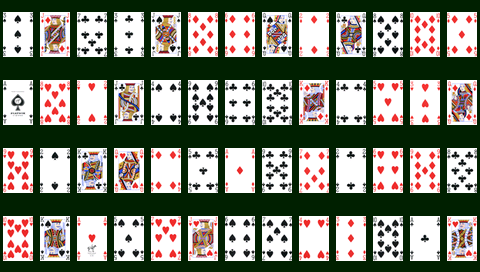ClearColorA(alpha)

Set clear value for the alpha channel

Parameters

alpha (int) – alpha value used when the color buffer is cleared. Range 0-255. The initial value is 0

This value is part of the graphics context and is saved and restored by SaveContext() and RestoreContext().

ClearColorRGB(red, green, blue)

Set clear values for red, green and blue channels

Parameters
• red (int) – red value used when the color buffer is cleared. Range 0-255. The initial value is 0

• green (int) – green value used when the color buffer is cleared. Range 0-255. The initial value is 0

• blue (int) – blue value used when the color buffer is cleared. Range 0-255. The initial value is 0

These values are part of the graphics context and are saved and restored by SaveContext() and RestoreContext().

Examples

gd.ClearColorRGB(0x00, 0x80, 0x80)         # teal
gd.Clear()
gd.ScissorSize(100, 200)
gd.ScissorXY(10, 20)
gd.ClearColorRGB(0xf8, 0x80, 0x17)         # orange
gd.Clear()Clear(c=1, s=1, t=1)

Clear buffers to preset values

Parameters
• c (int) – clear color buffer. Range 0-1

• s (int) – clear stencil buffer. Range 0-1

• t (int) – clear tag buffer. Range 0-1

Examples

gd.ClearColorRGB(0x00, 0x00, 0xff)  # Clear color to blue
gd.ClearStencil(0x80)               # Clear stencil to 0x80
gd.ClearTag(100)                    # Clear tag to 100
gd.Clear(1, 1, 1)                   # Go!ClearStencil(s)

Set clear value for the stencil buffer

Parameters

s (int) – value used when the stencil buffer is cleared. Range 0-255. The initial value is 0

This value is part of the graphics context and is saved and restored by SaveContext() and RestoreContext().

ClearTag(s)

Set clear value for the tag buffer

Parameters

s (int) – value used when the tag buffer is cleared. Range 0-255. The initial value is 0

This value is part of the graphics context and is saved and restored by SaveContext() and RestoreContext().

ColorA(alpha)

Set the current color alpha

Parameters

alpha (int) – alpha for the current color. Range 0-255. The initial value is 255

This value is part of the graphics context and is saved and restored by SaveContext() and RestoreContext().

Examples

gd.Begin(eve.POINTS)
gd.PointSize(24)
for i in range(0, 256, 5):
gd.ColorA(i)
gd.Vertex2f(2 * i, 136 + 120 * math.sin(i / 40))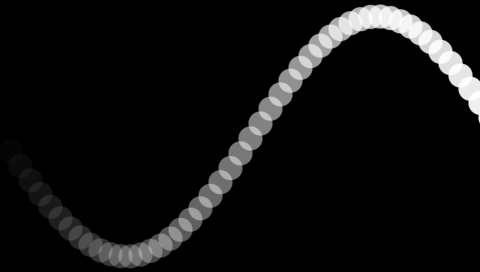Enable and disable writing of frame buffer color components

Parameters
• r (int) – allow updates to the frame buffer red component. Range 0-1. The initial value is 1

• g (int) – allow updates to the frame buffer green component. Range 0-1. The initial value is 1

• b (int) – allow updates to the frame buffer blue component. Range 0-1. The initial value is 1

• a (int) – allow updates to the frame buffer alpha component. Range 0-1. The initial value is 1

These values are part of the graphics context and are saved and restored by SaveContext() and RestoreContext().

Examples

gd.PointSize(270)
gd.Begin(eve.POINTS)
gd.ColorMask(1, 0, 0, 0)          # red only
gd.Vertex2f(240 - 100, 136)
gd.ColorMask(0, 1, 0, 0)          # green only
gd.Vertex2f(240, 136)
gd.ColorMask(0, 0, 1, 0)          # blue only
gd.Vertex2f(240 + 100, 136)ColorRGB(red, green, blue)

Set the drawing color

Parameters
• red (int) – red value for the current color. Range 0-255. The initial value is 255

• green (int) – green for the current color. Range 0-255. The initial value is 255

• blue (int) – blue for the current color. Range 0-255. The initial value is 255

These values are part of the graphics context and are saved and restored by SaveContext() and RestoreContext().

Examples

gd.Begin(eve.RECTS)
gd.ColorRGB(255, 128, 30)                  # orange
gd.Vertex2f(10, 10); gd.Vertex2f(470, 130)
gd.ColorRGB(0x4c, 0xc4, 0x17)              # apple green
gd.Vertex2f(10, 140); gd.Vertex2f(470, 260)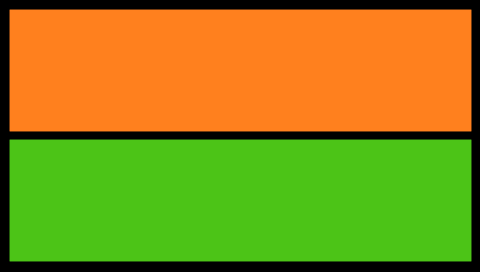End()

End drawing a graphics primitive

Vertex2ii() and Vertex2f() calls are ignored until the next Begin().

LineWidth(width)

Set the width of rasterized lines

Parameters

width (float) – line width in pixels. Range 0-511. The initial value is 1

This value is part of the graphics context and is saved and restored by SaveContext() and RestoreContext().

Examples

gd.Begin(eve.LINE_STRIP)
for x in range(0, 480, 40):
gd.LineWidth(x / 10)
gd.ColorRGB(0xff, random.randrange(256), random.randrange(256))
gd.Vertex2f(x, random.randrange(272))Macro(m)

Execute a single command from a macro register

Parameters

m (int) – macro register to read. Range 0-1

Nop()

No operation

Set the base address of the palette

Parameters

addr (int) – Address in graphics SRAM, 2-byte aligned. Range 0-4194303. The initial value is 0

This value is part of the graphics context and is saved and restored by SaveContext() and RestoreContext().

PointSize(size)

Set the diameter of rasterized points

Parameters

size (float) – point diameter in pixels. Range 0-1023. The initial value is 1

This value is part of the graphics context and is saved and restored by SaveContext() and RestoreContext().

Examples

gd.Begin(eve.POINTS)
for x in range(0, 480, 40):
gd.PointSize(x / 10)
gd.ColorRGB(0xff, random.randrange(256), random.randrange(256))
gd.Vertex2f(x, random.randrange(272))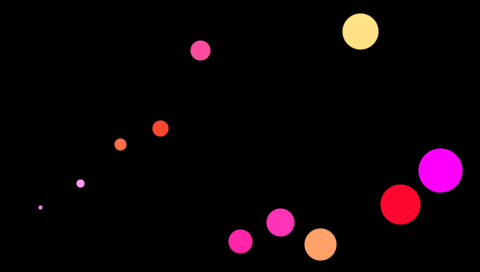RestoreContext()

Restore the current graphics context from the context stack

SaveContext()

Push the current graphics context on the context stack. The hardware’s graphics context stack is 4 levels deep.

Examples

gd.cmd_text(240,  64, 31, eve.OPT_CENTER, "WHITE")
gd.SaveContext()
gd.ColorRGB(0xff, 0x00, 0x00)
gd.cmd_text(240, 128, 31, eve.OPT_CENTER, "RED")
gd.RestoreContext()
gd.cmd_text(240, 196, 31, eve.OPT_CENTER, "WHITE AGAIN")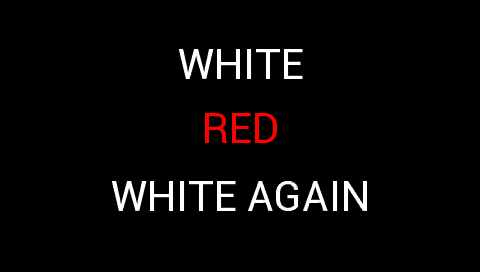ScissorSize(width, height)

Set the size of the scissor clip rectangle

Parameters
• width (int) – The width of the scissor clip rectangle, in pixels. Range 0-4095. The initial value is hsize

• height (int) – The height of the scissor clip rectangle, in pixels. Range 0-4095. The initial value is 2048

These values are part of the graphics context and are saved and restored by SaveContext() and RestoreContext().

Examples

gd.ScissorSize(400, 100)
gd.ScissorXY(35, 36)
gd.ClearColorRGB(0x00, 0x80, 0x80)
gd.Clear()
gd.cmd_text(240, 136, 31, eve.OPT_CENTER, "Scissor Example")
gd.ScissorXY(45, 140)
gd.ClearColorRGB(0xf8, 0x80, 0x17)
gd.Clear()
gd.cmd_text(240, 136, 31, eve.OPT_CENTER, "Scissor Example")ScissorXY(x, y)

Set the top left corner of the scissor clip rectangle

Parameters
• x (int) – The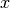coordinate of the scissor clip rectangle, in pixels. Range 0-2047. The initial value is 0

• y (int) – The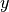coordinate of the scissor clip rectangle, in pixels. Range 0-2047. The initial value is 0

These values are part of the graphics context and are saved and restored by SaveContext() and RestoreContext().

Set function and reference value for stencil testing

Parameters

These values are part of the graphics context and are saved and restored by SaveContext() and RestoreContext().

Control the writing of individual bits in the stencil planes

Parameters

mask (int) – the mask used to enable writing stencil bits. Range 0-255. The initial value is 255

This value is part of the graphics context and is saved and restored by SaveContext() and RestoreContext().

StencilOp(sfail, spass)

Set stencil test actions

Parameters

These values are part of the graphics context and are saved and restored by SaveContext() and RestoreContext().

Examples

gd.StencilOp(eve.INCR, eve.INCR); # incrementing stencil
gd.PointSize(270)
gd.Begin(eve.POINTS)              # Draw three white circles
gd.Vertex2ii(240 - 100, 136)
gd.Vertex2ii(240, 136)
gd.Vertex2ii(240 + 100, 136)
gd.ColorRGB(0xff, 0x00, 0x00)     # Draw pixels with stencil==2 red
gd.StencilFunc(eve.EQUAL, 2, 255)
gd.Begin(eve.RECTS);              # Paint every pixel on the screen
gd.Vertex2f(0,0); gd.Vertex2f(480,272)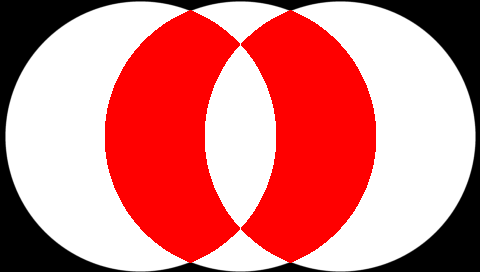Control the writing of the tag buffer

Parameters

mask (int) – allow updates to the tag buffer. Range 0-1. The initial value is 1

This value is part of the graphics context and is saved and restored by SaveContext() and RestoreContext().

Tag(s)

Set the current tag value

Parameters

s (int) – tag value. Range 0-255. The initial value is 255

This value is part of the graphics context and is saved and restored by SaveContext() and RestoreContext().

Vertex2f(x, y)

Draw a vertex. This operation draws a graphics primitive, depending on the primitive set by Begin().

Parameters
• x (float) – pixel x-coordinate

• y (float) – pixel y-coordinate

Vertex2ii(x, y, handle, cell)

Draw a vertex.

Parameters
• x (int) – x-coordinate in pixels. Range 0-511

• y (int) – y-coordinate in pixels. Range 0-511

• handle (int) – bitmap handle. Range 0-31

• cell (int) – cell number. Range 0-127

This method is an alternative to BitmapHandle(), Cell() and Vertex2f().

VertexFormat(frac)

Set the precision of coordinates used by Vertex2f()

Parameters

frac (int) – Number of fractional bits in X,Y coordinates. Range 0-7. The initial value is 4

This value is part of the graphics context and is saved and restored by SaveContext() and RestoreContext().

VertexTranslateX(x)

Set the vertex transformation’s x translation component

Parameters

x (float) – signed x-coordinate in pixels. Range ±4095. The initial value is 0

This value is part of the graphics context and is saved and restored by SaveContext() and RestoreContext().

VertexTranslateY(y)

Set the vertex transformation’s y translation component

Parameters

y (float) – signed y-coordinate in pixels. Range ±4095. The initial value is 0

This value is part of the graphics context and is saved and restored by SaveContext() and RestoreContext().

cmd_animdraw(ch)

Draw an animation

Parameters

ch (int) – animation channel

cmd_animframe(x, y, aoptr, frame)

Draw one animation frame

Parameters
• x (int) – x-coordinate

• y (int) – y-coordinate

• aoptr (int) – animation object pointer

• frame (int) – description

cmd_animframeram(x, y, aoptr, frame)

Draw one animation frame from RAM

Parameters
• x (int) – x-coordinate

• y (int) – y-coordinate

• aoptr (int) – animation object pointer

• frame (int) – description

cmd_animstart(ch, aoptr, loop)

Start an animation

Parameters
• ch (int) – animation channel

• aoptr (int) – animation object pointer

• loop (int) – description

cmd_animstartram(ch, aoptr, loop)

Start an animation from RAM

Parameters
• ch (int) – animation channel

• aoptr (int) – animation object pointer

• loop (int) – description

cmd_animstop(ch)

Stop playing an animation

Parameters

ch (int) – animation channel

cmd_animxy(ch, x, y)

Play an animation

Parameters
• ch (int) – animation channel

• x (int) – x-coordinate

• y (int) – y-coordinate

cmd_apilevel(level)

Set the API level

Parameters

level (int) – API level, 0 or 1.

API levelel. 0 is strict BT815 compatible, 1 is BT817.

Note

817 only

cmd_append(ptr, num)

Append main memory to the current display list

Parameters
• ptr (int) – address in EVE memory, 32-bit aligned

• num (int) – byte count, 32-bit aligned

Executes num bytes of drawing commands from graphics memory at ptr. This can be useful for using graphics memory as a cache for frequently used drawing sequences, much like OpenGL’s display lists.

cmd_appendf(ptr, num)

Append from flash to the current display list

Parameters
• ptr (int) – description

• num (int) – description

cmd_bgcolor(c)

Sets the widget background color

Parameters

c (int) – RGB color

cmd_bitmap_transform(x0, y0, x1, y1, x2, y2, tx0, ty0, tx1, ty1, tx2, ty2, result)

Computes an arbitrary bitmap transform

Parameters
• int (ty2) – point 0 screen x-coordinate

• int – point 0 screen y-coordinate

• int – point 1 screen x-coordinate

• int – point 1 screen y-coordinate

• int – point 2 screen x-coordinate

• int – point 2 screen y-coordinate

• int – point 0 bitmap x-coordinate

• int – point 0 bitmap y-coordinate

• int – point 1 bitmap x-coordinate

• int – point 1 bitmap y-coordinate

• int – point 2 bitmap x-coordinate

• int – point 2 bitmap y-coordinate

• result (int) – return code. Set to -1 on success.

cmd_button(x, y, w, h, font, options, s)

Draw a button with a text label

Parameters
• x (int) – button top left x

• y (int) – button top left y

• w (int) – button width in pixels

• h (int) – button height in pixels

• font (int) – font for label, 0-31

• options (int) – rendering options, see below

• s (str) – label text

The button command draws a button widget at screen (x, y) with pixel size w x h. label gives the text label.

The label is drawn centered within the button rectangle. It may cross multiple lines, separated by newline characters.

The following options may be logically-ored together:

Examples

gd.cmd_button(240 - 100, 136 - 40, 200, 80, 31, 0, "1 UP")cmd_calibrate(result)

Start the touch-screen calibration process

Parameters

result (int) – result code. Set to -1 on success.

cmd_calibratesub(x, y, w, h, result)

Start the touch-screen calibration process

Parameters
• x (int) – x-coordinate

• y (int) – y-coordinate

• w (int) – width

• h (int) – height

• result (int) – result code. Set to -1 on success.

Note

817 only

cmd_calllist(a)

Invoke a call list

Parameters

a (int) – call list pointer

Note

817 only

cmd_clearcache()

Clear the bitmap cache

cmd_clock(x, y, r, options, h, m, s, ms)

Draw a clock

Parameters
• x (int) – x-coordinate

• y (int) – y-coordinate

• r (int) – description

• options (int) – see below

• h (int) – height

• m (int) – description

• s (int) – description

• ms (int) – description

The following options may be logically-ored together:

Examples

gd.cmd_clock(240, 136, 120, 0, 8, 27, 13, 0)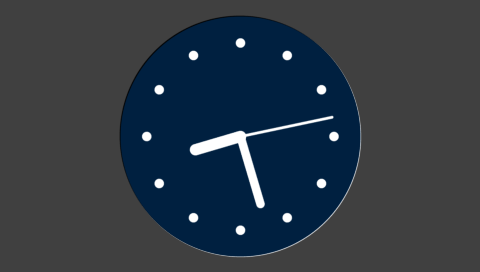gd.cmd_bgcolor(0x000000)
gd.ColorRGB(0x80, 0x80, 0x00)
gd.cmd_clock(240, 136, 120, eve.OPT_NOSECS | eve.OPT_FLAT, 1, 50, 0, 0)cmd_coldstart()

Reset all coprocessor state to its default values

cmd_crc(ptr)

Compute a CRC-32 for the currently displayed image

Parameters

ptr (int) – address in EVE memory

The 32-bit CRC is written to the given address.

cmd_dial(x, y, r, options, val)

Draws a dial, a circular widget with a single mark

Parameters
• x (int) – x-coordinate

• y (int) – y-coordinate

• r (int) – description

• options (int) – see below

• val (int) – value, 0-65535

The following options may be logically-ored together:

Examples

gd.cmd_dial(240, 136, 120, 0, 5333)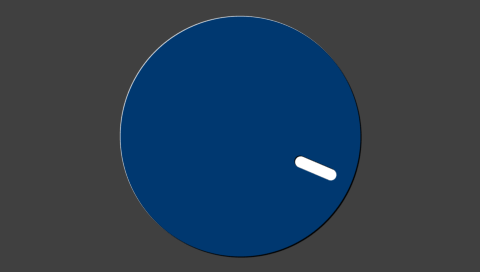cmd_dlstart()

Low-level command to start a new display list

cmd_endlist()

End a call list

Note

817 only

cmd_fgcolor(c)

Set the widget foreground color

Parameters

c (int) – 24-bit color

cmd_fillwidth(s)

Set the fill width used for multi-line text widgets

Parameters

s (int) – fill width in pixels

cmd_flashattach()

Attach to the flash

cmd_flashdetach()

Detach from flash

cmd_flasherase()

Perform a full-chip erase on the flash

cmd_flashfast(result)

Enter fast mode

Parameters

result (int) – result code. 0 on success.

cmd_flashprogram(dest, src, num)

Program flash from EVE memory

Parameters
• dest (int) – destination address in flash memory

• src (int) – source address in EVE memory

• num (int) – number of bytes to program

Parameters
• dest (int) – destination address in EVE memory

• src (int) – source address in flash memory

• num (int) – number of bytes to read

cmd_flashsource(ptr)

Set the flash source address for cmd_videostartf().

Parameters

ptr (int) – source address in flash memory

cmd_flashspidesel()

Deselect the flash

cmd_flashspirx(ptr, num)

Perform a raw SPI read from flash

Parameters
• ptr (int) – destination address in EVE memory

• num (int) – number of bytes to read

cmd_flashspitx(num!)

Perform a raw SPI write to flash

Parameters

int (num) – number of bytes to write

This command is followed by the num bytes of inline data.

cmd_flashupdate(dest, src, num)

Program flash from EVE memory

Parameters
• dest (int) – destination address in flash memory

• src (int) – source address in EVE memory

• num (int) – number of bytes to program

cmd_flashwrite(ptr, num)

Program flash from inline data

Parameters
• ptr (int) – destination address in flash memory

• int (num) – number of bytes to program

This command is followed by the num bytes of inline data.

cmd_fontcache(font, ptr, num)

Set up a font cache

Parameters
• font (int) – font number 0-31

• ptr (int) – start of font cache area

• num (int) – number of bytes to use for font cache

Note

817 only

cmd_fontcachequery(total, used)

Return statistics on font cache usage

Parameters
• total (int) – Total number of available bitmaps in the cache

• used (int) – Number of used bitmaps in the cache

cmd_gauge(x, y, r, options, major, minor, val, range)

Draw an indicator gauge

Parameters
• x (int) – x-coordinate

• y (int) – y-coordinate

• options (int) – see below

• major (int) – number of major tick marks

• minor (int) – number of minor tick marks

• val (int) – gauge value

• range (int) – range of gauge

Examples

gd.cmd_gauge(240, 136, 120, 0, 4, 2, 5333, 65535)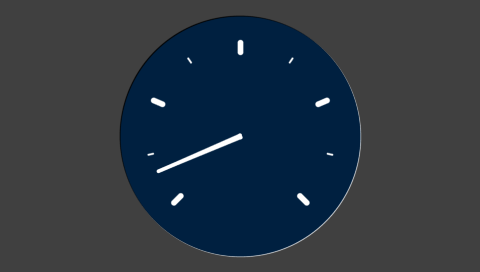cmd_getimage(source, fmt, w, h, palette)
Parameters
• source (int) – description

• fmt (int) – description

• w (int) – width

• h (int) – height

• palette (int) – description

cmd_getmatrix(a, b, c, d, e, f)

Returns the current bitmap transform matrix

Parameters
• a (int) – matrix coefficient

• b (int) – matrix coefficient

• c (int) – matrix coefficient

• d (int) – matrix coefficient

• e (int) – matrix coefficient

• f (int) – matrix coefficient

The matrix is returned as:

begin{bmatrix} a & b & c \ d & e & f \ end{bmatrix}

cmd_getprops(ptr, w, h)

Returns the parameters of the last loaded image

Parameters
• ptr (int) – bitmap source address

• w (int) – width

• h (int) – height

cmd_getptr(result)

Returns the first unallocated memory location

Parameters

result (int) – first unused address in EVE memory

set the 3D widget highlight color

Parameters

c (int) – a 24-bit color

cmd_gradient(x0, y0, rgb0, x1, y1, rgb1)

Draw a smooth color gradient between two points

Parameters
• int (rgb1) – point 0 x-coordinate

• int – point 0 y-coordinate

• int – a 24-bit color for point 0

• int – point 1 x-coordinate

• int – point 1 y-coordinate

• int – a 24-bit color for point 1

Examples

gd.cmd_gradient(0, 0,   0x0060c0, 0, 271, 0xc06000)
gd.cmd_text(240, 136, 31, eve.OPT_CENTER, "READY PLAYER ONE")cmd_gradienta(x0, y0, argb0, x1, y1, argb1)

Draw a smooth color gradient between two points with alpha transparency

Parameters
• int (argb1) – point 0 x-coordinate

• int – point 0 y-coordinate

• int – a 32-bit color for point 0

• int – point 1 x-coordinate

• int – point 1 y-coordinate

• int – a 32-bit color for point 1

cmd_hsf(w)

Configure the horizontal scanout filter

Parameters

w (int) – width in pixels

Note

817 only

cmd_inflate(ptr)

Decompress data into EVE memory

Parameters

int (ptr) – destination pointer in EVE memory

cmd_inflate2(ptr, options!)

Decompress data into EVE memory

Parameters
• ptr (int) – destination pointer in EVE memory

• int (options!) – options

cmd_interrupt(ms)

Trigger an interrupt

Parameters

ms (int) – delay before triggering interrupt in milliseconds

cmd_keys(x, y, w, h, font, options, s)

Draw a row of keys

Parameters
• x (int) – x-coordinate

• y (int) – y-coordinate

• w (int) – width

• h (int) – height

• font (int) – font number 0-31

• options (int) – see below

• s (str) – key labels

Examples

gd.cmd_keys(0, 136 - 40, 480, 80, 31, ord('u'), "qwertyuiop")Set the current bitmap transform matrix to the identity: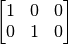Load an image into a bitmap

Parameters
• ptr (int) – destination address in EVE memory

• int (options) – options

This command is followed by the image data itself. Images may be in JPG or PNG format.

Examples

gd.cmd_loadimage(0, 0)
gd.Begin(eve.BITMAPS)
gd.Vertex2f(10, 72)Display the BridgeTek logo.

cmd_mediafifo(ptr, size)

Set the memory region used for the media FIFO

Parameters
• ptr (int) – start address in EVE memory

• size (int) – size of the media FIFO in bytes

cmd_memcpy(dest, src, num)

Copy EVE memory

Parameters
• dest (int) – destination address in EVE memory

• src (int) – source address in EVE memory

• num (int) – number of bytes to copy

cmd_memcrc(ptr, num, result)

Compute the CRC-32 of a region of EVE memory

Parameters
• ptr (int) – start address in EVE memory

• num (int) – size of region in bytes

• result (int) – address to write destination CRC in EVE memory

cmd_memset(ptr, value, num)

Set a region of EVE memory to a byte value

Parameters
• ptr (int) – destination address in EVE memory

• value (int) – byte value

• num (int) – size of region in bytes

cmd_memwrite(ptr, num)

Write the following inline data into EVE memory

Parameters
• ptr (int) – destination address in EVE memory

• int (num) – number of bytes to write

This command is followed by the inline data, and is padded to a 4-byte boundary. See cc() and align4().

cmd_memzero(ptr, num)

Set a region of EVE memory to zero

Parameters
• ptr (int) – destination address in EVE memory

• num (int) – size of region in bytes

cmd_newlist(a)

Start compiling a call list

Parameters

a (int) – call list pointer

Note

817 only

cmd_nop()

No operation.

cmd_number(x, y, font, options, n)

Draw a number

Parameters
• x (int) – x-coordinate

• y (int) – y-coordinate

• font (int) – font number 0-31

• options (int) – see below

• n (int) – number

renders a number n in font font at screen (x, y). If an integer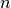is supplied as an option, then leading zeroes are added so thatdigits are always drawn.

The following options may be logically-ored together:

See also cmd_setbase()

Examples

gd.cmd_number(240, 45, 31, eve.OPT_CENTER, 42)
gd.cmd_number(240, 136, 31, eve.OPT_CENTER | 4, 42)
gd.cmd_number(240, 226, 31, eve.OPT_CENTER | 8, 42)cmd_pclkfreq(ftarget, rounding, factual)

Set the PCLK frequency

Parameters
• ftarget (int) – target frequency, in Hz

• rounding (int) – rounding mode

• factual (int) – return value, actual frequency

Note

817 only

cmd_playvideo(options!)

Play a video from inline video data

Parameters

int (options) – playback options

cmd_progress(x, y, w, h, options, val, range)

Draw a progress bar

Parameters
• x (int) – x-coordinate

• y (int) – y-coordinate

• w (int) – width

• h (int) – height

• options (int) – see below

• val (int) – progress bar value

• range (int) – progress bar range

Examples

gd.cmd_progress(10, 136, 460, 10, 0, 25333, 65535)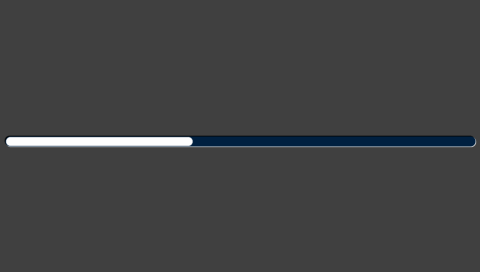The following options may be logically-ored together:

Reads a 32-bit value from EVE memory

Parameters
• ptr (int) – source address in EVE memory

• result (int) – register value

cmd_regwrite(ptr, val)

Writes a 32-bit value to EVE memory

Parameters
• ptr (int) – address in EVE memory

• val (int) – 32-bit value

cmd_resetfonts()

Reset all ROM fonts (numbers 16-31) to their default settings

cmd_return()

Return from a Call List

Note

817 only

cmd_romfont(font, romslot)

Load a ROM font into a font handle

Parameters
• font (int) – font number 0-31

• romslot (int) – ROM font number 16-34

cmd_rotate(a)

Apply a rotation to the bitmap transform matrix

Parameters

a (float) – clockwise rotation angle, in degrees

cmd_rotatearound(x, y, a, s)

Apply a rotation and scale to the bitmap transform matrix around a given point

Parameters
• x (int) – center of rotation x-coordinate

• y (int) – center of rotation y-coordinate

• a (float) – clockwise rotation angle, in degrees

• s (float) – scale factor

Run all active animations

Parameters

• play (int) – y-coordinate

Note

817 only

cmd_scale(sx, sy)

Apply a scale to the bitmap transform matrix

Parameters
• sx (float) – x-axis scale factor

• sy (float) – y-axis scale factor

cmd_screensaver()

Run the screen-saver function. Use cmd_stop() to stop it.

cmd_scrollbar(x, y, w, h, options, val, size, range)

Draw a scroll bar

Parameters
• x (int) – x-coordinate

• y (int) – y-coordinate

• w (int) – width

• h (int) – height

• options (int) – see below

• val (int) – bar starting position

• size (int) – bar size

• range (int) – range of entire bar

The following options may be logically-ored together:

Examples

gd.cmd_scrollbar(30, 136, 420, 30, 0, 25333, 10000, 65535)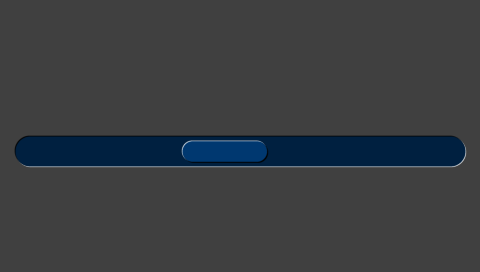cmd_setbase(b)

Set the base used by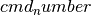. The default base is 10 (decimal)

Parameters

b (int) – base, 1-36

cmd_setbitmap(source, fmt, w, h)

Set all the parameters for a bitmap.

Parameters
cmd_setfont(font, ptr)

Load a font slot from a font in RAM

Parameters
• font (int) – font number 0-31

• ptr (int) – address to the font register value

cmd_setfont2(font, ptr, firstchar)

Load a font slot from a font in RAM

Parameters
• font (int) – font number 0-31

• ptr (int) – address of the font descriptor block in EVE memory

• firstchar (int) – first valid character in font

cmd_setmatrix()

Append the current transform matrix to the display list.

cmd_setrotate(r)

Change screen orientation by setting REG_ROTATE and adjusting the touch transform matrix.

Parameters

r (int) – new orientation

cmd_setscratch(handle)

Set the bitmap handle used for widget drawing. The default handle is 15.

Parameters

handle (int) – bitmap handle

cmd_sketch(x, y, w, h, ptr, format)

Begin sketching. Use cmd_stop() to stop it.

Parameters
• x (int) – x-coordinate

• y (int) – y-coordinate

• w (int) – width

• h (int) – height

• ptr (int) – bitmap start address

• format (int) – either L1 or L8

cmd_slider(x, y, w, h, options, val, range)

Draw a slider

Parameters
• x (int) – x-coordinate

• y (int) – y-coordinate

• w (int) – width

• h (int) – height

• options (int) – see below

• val (int) – position of slider knob

• range (int) – range of entire slider

The following options may be logically-ored together:

Examples

gd.cmd_slider(30, 136, 420, 30, 0, 25333, 65535)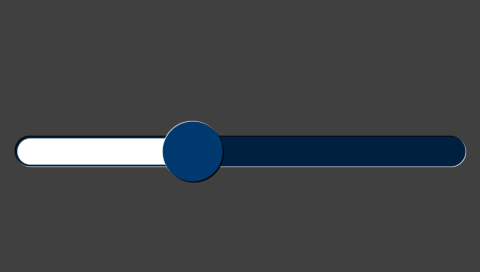cmd_snapshot(ptr)

Write a snapshot of the current screen as a bitmap

Parameters

ptr (int) – destination bitmap address in EVE memory

cmd_snapshot2(fmt, ptr, x, y, w, h)

Write a snapshot of the current screen as a bitmap

Parameters
• fmt (int) – bitmap format

• ptr (int) – destination bitmap address in EVE memory

• x (int) – x-coordinate

• y (int) – y-coordinate

• w (int) – width of snapshot rectangle

• h (int) – height of snapshot rectangle

cmd_spinner(x, y, style, scale)

Display a “waiting” spinner Use cmd_stop() to stop it.

Parameters
• x (int) – x-coordinate

• y (int) – y-coordinate

• style (int) – see below

• scale (int) – element size. 0 is small, 1 medium, 2 huge.

There are four spinner styles available:

• 0 circular

• 1 linear

• 2 clock

• 3 rotating disks

Examples

gd.cmd_spinner(240, 136, 0, 1)cmd_stop()

Stop any currently running background tasks.

cmd_swap()

Low-level command to swap the display lists

cmd_sync()

Delay execution until the next vertical blanking interval

cmd_testcard()

Draw a diagnostic test-card

Note

817 only

cmd_text(x, y, font, options, s)

Draws text

Parameters
• x (int) – x-coordinate

• y (int) – y-coordinate

• font (int) – font number 0-31

• options (int) – see below

• s (str) – text

renders a number n in font font at screen (x, y).

The following options may be logically-ored together:

Examples

ipsum = 'Lorem ipsum dolor sit amet, consectetur adipiscing elit, sed do eiusmod tempor incididunt ut labore et dolore magna aliqua. Ut enim ad minim veniam, quis nostrud exercitation ullamco laboris'
gd.cmd_fillwidth(400)
gd.ColorRGB(0xf8, 0x80, 0x17) # orange
gd.cmd_text(40, 10, 30, eve.OPT_FILL, ipsum)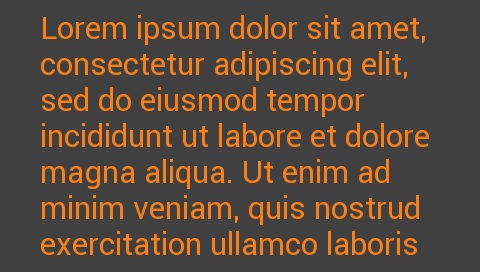gd.ColorRGB(0x00, 0x00, 0x00)
gd.Begin(eve.LINES)
gd.Vertex2f(240, 0)
gd.Vertex2f(240, gd.h)
gd.ColorRGB(0xff, 0xff, 0xff)
gd.cmd_text(240, 50, 29, 0, "default")
gd.cmd_text(240,100, 29, eve.OPT_RIGHTX, "eve.OPT_RIGHTX")
gd.cmd_text(240,150, 29, eve.OPT_CENTERX, "eve.OPT_CENTERX")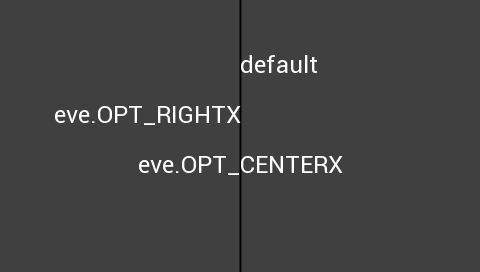t = 31.09
gd.cmd_text(240,100, 29, eve.OPT_FORMAT | eve.OPT_CENTER,
"Temperature is %d.%02d C", int(t), int(t * 100) % 100)cmd_toggle(x, y, w, font, options, state, s1, s0)

Draws a toggle widget

Parameters
• x (int) – x-coordinate

• y (int) – y-coordinate

• w (int) – width

• font (int) – font number 0-31

• options (int) – see below

• state (int) – toggle position 0-65535

• s1 (str) – label for right side

• s0 (str) – label for left side

Examples

gd.cmd_toggle(180,  20, 120, 31, 0, 0,     "yes", "no")
gd.cmd_toggle(180, 120, 120, 31, 0, 32768, "yes", "no")
gd.cmd_toggle(180, 220, 120, 31, 0, 65535, "yes", "no")gd.cmd_text(gd.w // 2, 100, 30, eve.OPT_CENTER, "What is your age, Liesl?")
gd.cmd_toggle(180, 160, 120, 31, eve.OPT_FORMAT, 32768, "%d", "%d", 17, 16)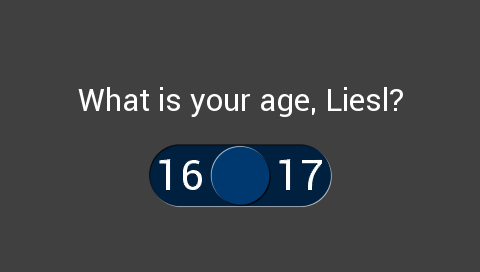The following options may be logically-ored together:

cmd_track(x, y, w, h, tag)

Start tracking touches for a graphical object

Parameters
• x (int) – x-coordinate

• y (int) – y-coordinate

• w (int) – width

• h (int) – height

• tag (int) – object tag number 0-255

Up to 255 objects may be tracked. Each object may be either linear (if either width or height is 1) or rotary (if both width and height are 1).

cmd_translate(tx, ty)

Apply a translation to the bitmap transform matrix

Parameters
• tx (int) – translation in x-axis

• ty (int) – translation in y-axis

cmd_videoframe(dst, ptr)

Decode a single video frame

Parameters
• dst (int) – bitmap destination in EVE memory

• ptr (int) – completion flag address in EVE memory

cmd_videostart()

Start video playback

cmd_videostartf()

Start video playback

cmd_wait(us)

Wait

Parameters

us (int) – wait duration in microseconds

Note

817 only

cc(b)

Append bytes to the command FIFO.

Parameters

b (bytes) – The bytes to add. Its length must be a multiple of 4.

finish()

Send any queued drawing commands directly to the hardware, and return after they have all completed execution.

flush()

Send any queued drawing commands directly to the hardware.

swap()

End the current display list and dispatch it to the graphics hardware. Start compiling the display list for the next frame.

screenshot_im()

Return the current screen contents as an image

Returns image

Image of the current screen

The returned image is a PIL Image with mode RGB and size (w, h).

It can be saved to a file with:

gd.screenshot_im().save("screenshot.png")


Note

available on PC only

# Module constants¶

NEVER = 0
LESS = 1
LEQUAL = 2
GREATER = 3
GEQUAL = 4
EQUAL = 5
NOTEQUAL = 6
ALWAYS = 7

RED = 2
GREEN = 3
BLUE = 4
ALPHA = 5

## Bitmap Formats used by EVE.BitmapLayout() and EVE.cmd_setbitmap()¶

ARGB1555 = 0
L1 = 1
L4 = 2
L8 = 3
RGB332 = 4
ARGB2 = 5
ARGB4 = 6
RGB565 = 7
PALETTED = 8
TEXT8X8 = 9
TEXTVGA = 10
BARGRAPH = 11
PALETTED565 = 14
PALETTED4444 = 15
PALETTED8 = 16
L2 = 17
GLFORMAT = 31
ASTC_4x4 = 0x93B0
ASTC_5x4 = 0x93B1
ASTC_5x5 = 0x93B2
ASTC_6x5 = 0x93B3
ASTC_6x6 = 0x93B4
ASTC_8x5 = 0x93B5
ASTC_8x6 = 0x93B6
ASTC_8x8 = 0x93B7
ASTC_10x5 = 0x93B8
ASTC_10x6 = 0x93B9
ASTC_10x8 = 0x93BA
ASTC_10x10 = 0x93BB
ASTC_12x10 = 0x93BC
ASTC_12x12 = 0x93BD

NEAREST = 0
BILINEAR = 1

BORDER = 0
REPEAT = 1

KEEP = 1
REPLACE = 2
INCR = 3
DECR = 4
INVERT = 5

## Blend factors for BlendFunc()¶

ZERO = 0
ONE = 1
SRC_ALPHA = 2
DST_ALPHA = 3
ONE_MINUS_SRC_ALPHA = 4
ONE_MINUS_DST_ALPHA = 5

BITMAPS = 1
POINTS = 2
LINES = 3
LINE_STRIP = 4
EDGE_STRIP_R = 5
EDGE_STRIP_L = 6
EDGE_STRIP_A = 7
EDGE_STRIP_B = 8
RECTS = 9

## Options bitfields¶

OPT_MONO = 1
OPT_NODL = 2
OPT_FLAT = 256
OPT_CENTERX = 512
OPT_CENTERY = 1024
OPT_CENTER = 1536
OPT_NOBACK = 4096
OPT_NOTICKS = 8192
OPT_NOHM = 16384
OPT_NOPOINTER = 16384
OPT_NOSECS = 32768
OPT_NOHANDS = 49152
OPT_RIGHTX = 2048
OPT_SIGNED = 256
OPT_FULLSCREEN = 8
OPT_MEDIAFIFO = 16
OPT_FORMAT = 4096
OPT_FILL = 8192

## Sample formats for use with REG_PLAYBACK_FORMAT¶

LINEAR_SAMPLES = 0
ULAW_SAMPLES = 1

## Instrument names for use with REG_SOUND¶

HARP = 0x40
XYLOPHONE = 0x41
TUBA = 0x42
GLOCKENSPIEL = 0x43
ORGAN = 0x44
TRUMPET = 0x45
PIANO = 0x46
CHIMES = 0x47
MUSICBOX = 0x48
BELL = 0x49
CLICK = 0x50
SWITCH = 0x51
COWBELL = 0x52
NOTCH = 0x53
HIHAT = 0x54
KICKDRUM = 0x55
POP = 0x56
CLACK = 0x57
CHACK = 0x58
MUTE = 0x60
UNMUTE = 0x61

RAM_CMD = 0x308000
RAM_DL = 0x300000
REG_CLOCK = 0x302008
REG_CMDB_SPACE = 0x302574
REG_CMDB_WRITE = 0x302578
REG_CMD_DL = 0x302100
REG_CMD_WRITE = 0x3020fc
REG_CPURESET = 0x302020
REG_DITHER = 0x302060
REG_DLSWAP = 0x302054
REG_FRAMES = 0x302004
REG_FREQUENCY = 0x30200c
REG_GPIO = 0x302094
REG_GPIO_DIR = 0x302090
REG_HCYCLE = 0x30202c
REG_HOFFSET = 0x302030
REG_HSIZE = 0x302034
REG_HSYNC0 = 0x302038
REG_HSYNC1 = 0x30203c
REG_ID = 0x302000
REG_INT_EN = 0x3020ac
REG_INT_FLAGS = 0x3020a8
REG_MACRO_0 = 0x3020d8
REG_MACRO_1 = 0x3020dc
REG_OUTBITS = 0x30205c
REG_PCLK = 0x302070
REG_PCLK_POL = 0x30206c
REG_PLAY = 0x30208c
REG_PLAYBACK_FORMAT = 0x3020c4
REG_PLAYBACK_FREQ = 0x3020c0
REG_PLAYBACK_LENGTH = 0x3020b8
REG_PLAYBACK_LOOP = 0x3020c8
REG_PLAYBACK_PLAY = 0x3020cc
REG_PLAYBACK_START = 0x3020b4
REG_PWM_DUTY = 0x3020d4
REG_PWM_HZ = 0x3020d0
REG_ROTATE = 0x302058
REG_SOUND = 0x302088
REG_SWIZZLE = 0x302064
REG_TAG = 0x30207c
REG_TAG_X = 0x302074
REG_TAG_Y = 0x302078
REG_TAP_CRC = 0x302024
REG_TOUCH_CHARGE = 0x30210c
REG_TOUCH_DIRECT_XY = 0x30218c
REG_TOUCH_DIRECT_Z1Z2 = 0x302190
REG_TOUCH_MODE = 0x302104
REG_TOUCH_OVERSAMPLE = 0x302114
REG_TOUCH_RAW_XY = 0x30211c
REG_TOUCH_RZ = 0x302120
REG_TOUCH_RZTHRESH = 0x302118
REG_TOUCH_SCREEN_XY = 0x302124
REG_TOUCH_SETTLE = 0x302110
REG_TOUCH_TAG = 0x30212c
REG_TOUCH_TAG_XY = 0x302128
REG_TOUCH_TRANSFORM_A = 0x302150
REG_TOUCH_TRANSFORM_B = 0x302154
REG_TOUCH_TRANSFORM_C = 0x302158
REG_TOUCH_TRANSFORM_D = 0x30215c
REG_TOUCH_TRANSFORM_E = 0x302160
REG_TOUCH_TRANSFORM_F = 0x302164
REG_TRACKER = 0x309000
REG_TRIM = 0x302180
REG_VCYCLE = 0x302040
REG_VOFFSET = 0x302044
REG_VOL_PB = 0x302080
REG_VOL_SOUND = 0x302084
REG_VSIZE = 0x302048
REG_VSYNC0 = 0x30204c
REG_VSYNC1 = 0x302050
REG_MEDIAFIFO_BASE = 0x30901c